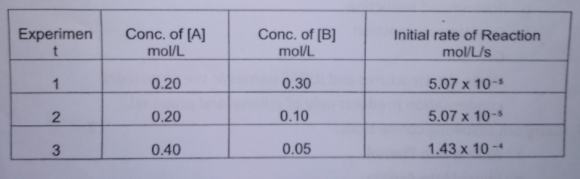# Problem: Find the order of the reaction with respect to A and B and find the rate law expression for:2 A + B → C

###### FREE Expert Solution

We’re being asked to find the order of the reaction with respect to A and B and find the rate law expression for:

2 A + B → C

Recall that the rate law only focuses on the reactant concentrations and has a general form of:

k = rate constant
A & B = reactants
x & y = reactant orders

We’re going to calculate the rate law and the rate constant using the following steps:

86% (257 ratings)###### Problem Details

Find the order of the reaction with respect to A and B and find the rate law expression for:

2 A + B → C